Using SAMBA

10 February, 2020

In this vignette, we provide a brief introduction to using the R package SAMBA (selection and misclassification bias adjustment). The purpose of this package is to provide resources for fitting a logistic regression for a binary outcome in the presence of outcome misclassification (in particular, imperfect sensitivity) along with potential selection bias. We will not include technical details about this estimation approach and instead focus on implementation. For additional details about the estimation algorithm, we refer the reader to Statistical inference for association studies using electronic health records: handling both selection bias and outcome misclassification’’ by Lauren J Beesley and Bhramar Mukherjee on medRvix.

Model structure

Let binary $$D$$ represent a patient’s true disease status for a disease of interest, e.g. diabetes. Suppose we are interested in the relationship between $$D$$ and and person-level information, $$Z$$. $$Z$$ may contain genetic information, lab results, age, gender, or any other characteristics of interest. We call this relationship the disease mechanism as in Figure 1.

Suppose we consider a large health care system-based database with the goal of making inference about some defined general population. Let $$S$$ indicate whether a particular subject in the population is sampled into our dataset (for example, by going to a particular hospital and consenting to share biosamples), where the probability of an individual being included in the current dataset may depend on the underlying lifetime disease status, $$D$$, along with additional covariates, $$W$$. $$W$$ may also contain some or all adjustment factors in $$Z$$. In the EHR setting, we may often expect the sampled and non-sampled patients to have different rates of the disease, and other factors such as patient age, residence, access to care and general health state may also impact whether patients are included in the study base or not. We will call this mechanism the selection mechanism.

Instances of the disease are recorded in hospital or administrative records. We might expect factors such as the patient age, the length of follow-up, and the number of hospital visits to impact whether we actually observe/record the disease of interest for a given person. Let $$D^*$$ be the observed disease status. $$D^*$$ is a potentially misclassified version of $$D$$. We will call the mechanism generating $$D^*$$ the observation mechanism. We will assume that misclassification is primarily through underreporting of disease. In other words, we will assume that $$D^*$$ has perfect specificity and potentially imperfect sensitivity with respect to $$D$$. Let $$X$$ denote patient and provider-level predictors related to the true positive rate (sensitivity).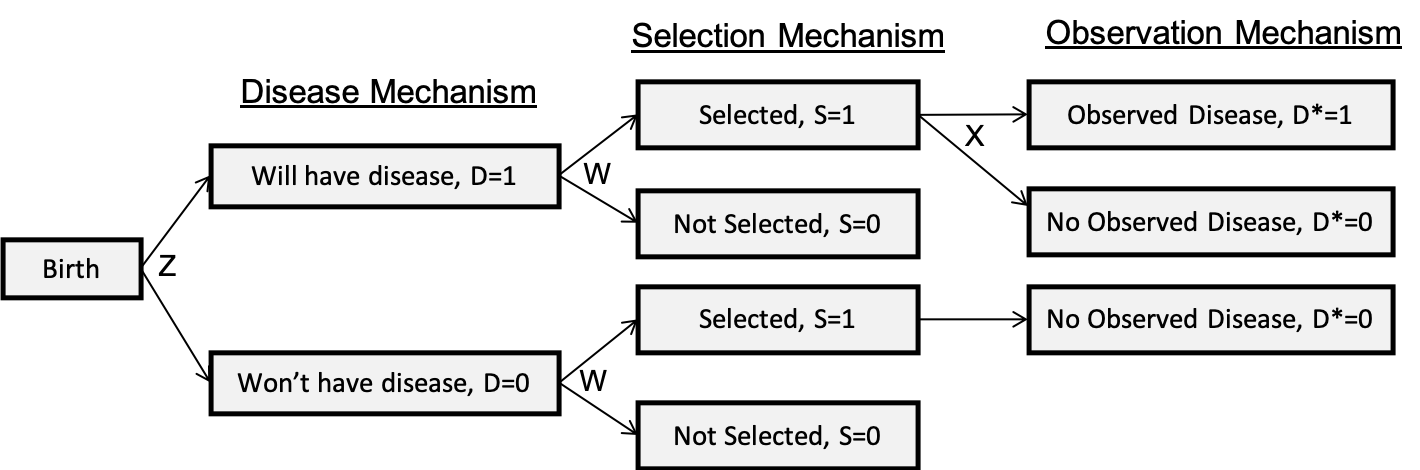Figure 1: Model Structure

We express the conceptual model as follows:

$\text{Disease Model}: \text{logit}(P(D=1 \vert Z; \theta )) = \theta_0 + \theta_Z Z$ $\text{Selection Model}: P(S=1 \vert D, W; \phi )$ $\text{Sensitivity/Observation Model}: \text{logit}(P(D^*=1 \vert D=1, S=1, X; \beta)) = \beta_0 + \beta_X X$

Simulate data

We start our exploration by simulating some binary data subject to misclassification of the outcome and selection bias. Variables related to selection are D and W. Variables related to sensitivity are X. Variables of interest are Z, which are related to W and X. In this simulation, W is also independently related to D.

library(SAMBA)
library(MASS)
expit <- function(x) exp(x) / (1 + exp(x))
logit <- function(x) log(x / (1 - x))

nobs <- 5000

### Generate Predictors and Follow-up Information
set.seed(1234)
cov <- mvrnorm(n = nobs, mu = rep(0, 3), Sigma = rbind(c(1,   0, 0.4),
c(0,   1,   0),
c(0.4, 0,   1)))

data <- data.frame(Z = cov[, 1], X = cov[, 2], W = cov[, 3])

# Generate random uniforms
set.seed(5678)
U1 <- runif(nobs)
set.seed(4321)
U2 <- runif(nobs)
set.seed(8765)
U3 <- runif(nobs)

# Generate Disease Status
DISEASE <- expit(-2 + 0.5 * data$Z) data$D   <- ifelse(DISEASE > U1, 1, 0)

# Relate W and D
data$W <- data$W + 1 * data$D # Generate Misclassification SENS <- expit(-0.4 + 1 * data$X)
SENS[data$D == 0] = 0 data$Dstar <- ifelse(SENS > U2, 1, 0)

# Generate Sampling Status
SELECT <- expit(-0.6 + 1 * data$D + 0.5 * data$W)
S  <- ifelse(SELECT > U3, T, F)

# Observed Data
data.samp <- data[S,]

# True marginal sampling ratio
prob1 <- expit(-0.6 + 1 * 1 + 0.5 * data$W) prob0 <- expit(-0.6 + 1 * 0 + 0.5 * data$W)
r.marg.true <- mean(prob1[data$D == 1]) / mean(prob0[data$D == 0])

# True inverse probability of sampling weights
prob.WD <- expit(-0.6 + 1 * data.samp$D + 0.5 * data.samp$W)
weights <- nrow(data.samp) * (1  / prob.WD) / (sum(1 / prob.WD))

# True associations with D in population
trueX <- glm(D ~ X, binomial(), data = data)
trueZ <- glm(D ~ Z, binomial(), data = data)

# Initial Parameter Values
fitBeta  <- glm(Dstar ~ X, binomial(), data = data.samp)
fitTheta <- glm(Dstar ~ Z, binomial(), data = data.samp)

Estimating sensitivity

In our paper, we develop several strategies for estimating either marginal sensitivity or sensitivity as a function of covariates X. Here, we apply several of the proposed strategies.

# Using marginal sampling ratio r and P(D=1)
sens1 <- sensitivity(data.samp$Dstar, data.samp$X, mean(data$D), r = r.marg.true) # Using inverse probability of selection weights and P(D=1) sens2 <- sensitivity(data.samp$Dstar, data.samp$X, prev = mean(data$D),
weights = weights)

# Using marginal sampling ratio r and P(D=1|X)
prev  <- predict(trueX, newdata = data.samp, type = 'response')
sens3 <- sensitivity(data.samp$Dstar, data.samp$X, prev, r = r.marg.true)

# Using inverse probability of selection weights and P(D=1|X)
prev  <- predict(trueX, newdata = data.samp, type = 'response')
sens4 <- sensitivity(data.samp$Dstar, data.samp$X, prev, weights = weights)

Estimating log-odds ratio for D|Z

We propose several strategies for estimating the association between D and Z from a logistic regression model in the presence of different biasing factors. Below, we provide code for implementing these methods.

# Approximation of D*|Z
approx1 <- approxdist(data.samp$Dstar, data.samp$Z, sens1$c_marg, weights = weights) # Non-logistic link function method nonlog1 <- nonlogistic(data.samp$Dstar, data.samp$Z, c_X = sens3$c_X,
weights = weights)

# Direct observed data likelihood maximization without fixed intercept
start <- c(coef(fitTheta), logit(sens1$c_marg), coef(fitBeta)) fit1 <- obsloglik(data.samp$Dstar, data.samp$Z, data.samp$X, start = start,
weights = weights)
obsloglik1 <- list(param = fit1$param, variance = diag(fit1$variance))

# Direct observed data likelihood maximization with fixed intercept
fit2   <- obsloglik(data.samp$Dstar, data.samp$Z, data.samp$X, start = start, beta0_fixed = logit(sens1$c_marg), weights = weights)
obsloglik2 <- list(param = fit2$param, variance = diag(fit2$variance))

# Expectation-maximization algorithm without fixed intercept
fit3 <- obsloglikEM(data.samp$Dstar, data.samp$Z, data.samp$X, start = start, weights = weights) obsloglik3 <- list(param = fit3$param, variance = diag(fit3$variance)) # Expectation-maximization algorithm with fixed intercept fit4 <- obsloglikEM(data.samp$Dstar, data.samp$Z, data.samp$X, start = start,
beta0_fixed = logit(sens1$c_marg), weights = weights) obsloglik4 <- list(param = fit4$param, variance = diag(fit4\$variance))

Plotting sensitivity estimates

Figure 2 shows the estimated individual-level sensitivity values when the marginal sampling ratio (r-tilde) is correctly specified. We can see that there is strong concordance with the true sensitivity values.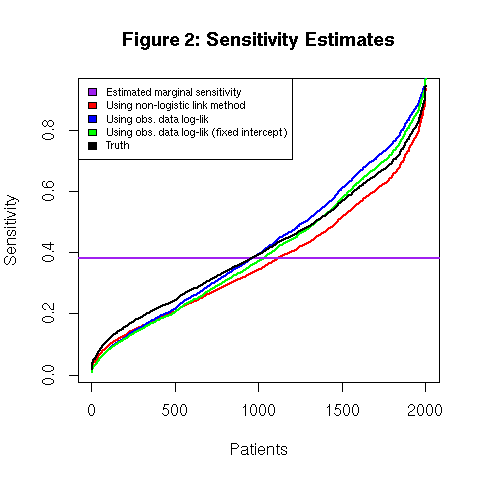Figure 3 shows the estimated individual-level sensitivity values across different marginal sampling ratio values. In reality, we will rarely know the truth, and this strategy can help us obtain reasonable values for sensitivity across plausible sampling ratio values.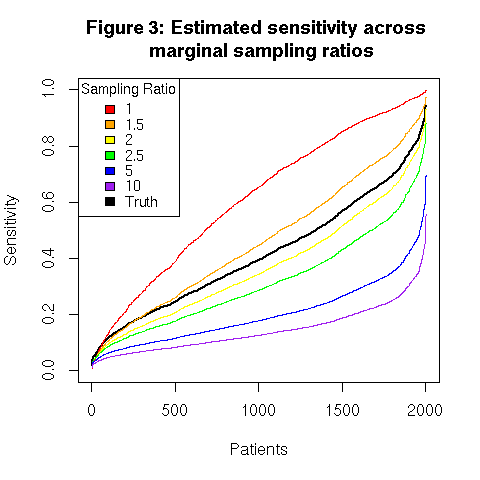Evaluating observed data log-likelihood maximization

Figure 4 shows the maximized log-likelihood values for fixed values of $$\beta_0$$ obtained using direct maximization of the observed data log-likelihood. The provide likelihood does an excellent job at recovering the true value of $$\beta_0$$.

Figure 5 shows the log-likelihood values across iterations of the expectation-maximization algorithm estimation method when we do and do not fix $$\beta_0$$. We see faster and cleaner convergence to the MLE when we fix the intercept $$\beta_0$$.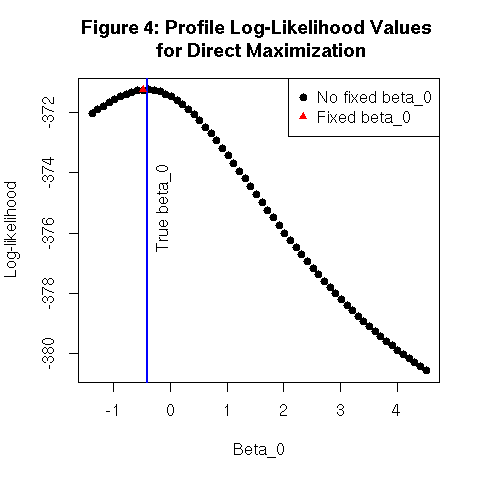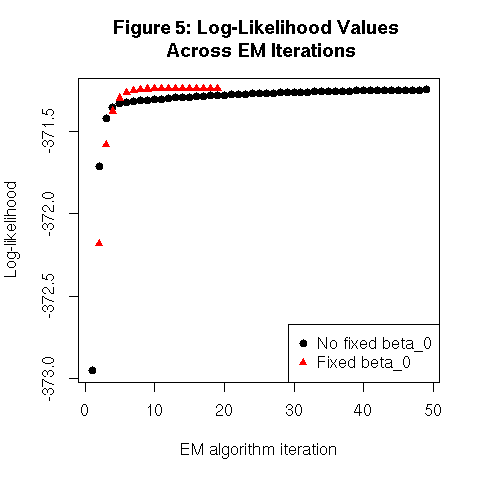Plotting log-odds ratio estimates

Figure 6 shows the estimated log-odds ratio relating $$D$$ and $$Z$$ for the various analysis methods. Uncorrected (complete case with misclassified outcome) analysis produces bias, and some methods reduce this bias. Recall, this is a single simulated dataset, and the corrected estimators may not always equal the truth for a given simulation. When $$W$$ and $$D$$ are independently associated, the method using the approximated $$D^* \vert Z$$ relationship and marginal sensitivity can sometimes perform poorly.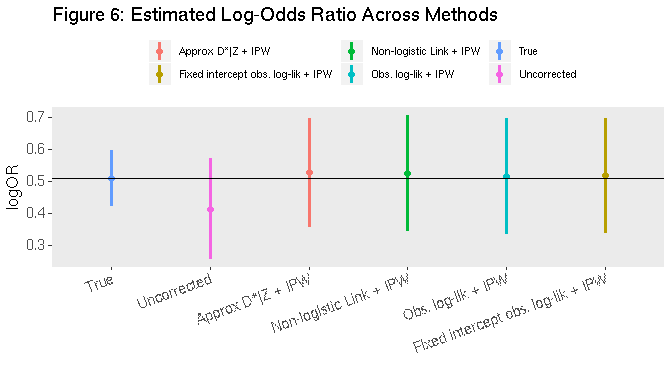Reference

Statistical inference for association studies using electronic health records: handling both selection bias and outcome misclassification Lauren J Beesley and Bhramar Mukherjee medRxiv 2019.12.26.19015859